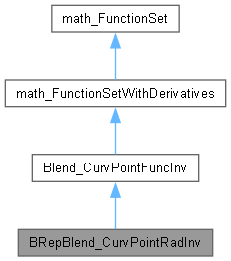Function of reframing between a point and a curve. valid in cases of constant and progressive radius. This function is used to find a solution on a done point of the curve 1 when using RstRstConsRad or CSConstRad... The vector <X> used in Value, Values and Derivatives methods has to be the vector of the parametric coordinates w, U where w is the parameter on the guide line, U are the parametric coordinates of a point on the partner curve 2. More...

`#include <BRepBlend_CurvPointRadInv.hxx>`[legend]

## Public Member Functions

void Set (const Standard_Integer Choix)

Standard_Integer NbEquations () const
returns 2. More...

Standard_Boolean Value (const math_Vector &X, math_Vector &F)
computes the values <F> of the Functions for the variable <X>. Returns True if the computation was done successfully, False otherwise. More...

Standard_Boolean Derivatives (const math_Vector &X, math_Matrix &D)
returns the values <D> of the derivatives for the variable <X>. Returns True if the computation was done successfully, False otherwise. More...

Standard_Boolean Values (const math_Vector &X, math_Vector &F, math_Matrix &D)
returns the values <F> of the functions and the derivatives <D> for the variable <X>. Returns True if the computation was done successfully, False otherwise. More...

void Set (const gp_Pnt &P)
Set the Point on which a solution has to be found. More...

void GetTolerance (math_Vector &Tolerance, const Standard_Real Tol) const
Returns in the vector Tolerance the parametric tolerance for each of the 3 variables; Tol is the tolerance used in 3d space. More...

void GetBounds (math_Vector &InfBound, math_Vector &SupBound) const
Returns in the vector InfBound the lowest values allowed for each of the 3 variables. Returns in the vector SupBound the greatest values allowed for each of the 3 variables. More...

Standard_Boolean IsSolution (const math_Vector &Sol, const Standard_Real Tol)
Returns Standard_True if Sol is a zero of the function. Tol is the tolerance used in 3d space. More...Public Member Functions inherited from Blend_CurvPointFuncInv
Standard_Integer NbVariables () const
Returns 3. More...Public Member Functions inherited from math_FunctionSet
virtual Standard_Integer GetStateNumber ()
Returns the state of the function corresponding to the latestcall of any methods associated with the function. This function is called by each of the algorithms described later which define the function Integer Algorithm::StateNumber(). The algorithm has the responsibility to call this function when it has found a solution (i.e. a root or a minimum) and has to maintain the association between the solution found and this StateNumber. Byu default, this method returns 0 (which means for the algorithm: no state has been saved). It is the responsibility of the programmer to decide if he needs to save the current state of the function and to return an Integer that allows retrieval of the state. More...

virtual ~math_FunctionSet ()

## Detailed Description

Function of reframing between a point and a curve. valid in cases of constant and progressive radius. This function is used to find a solution on a done point of the curve 1 when using RstRstConsRad or CSConstRad... The vector <X> used in Value, Values and Derivatives methods has to be the vector of the parametric coordinates w, U where w is the parameter on the guide line, U are the parametric coordinates of a point on the partner curve 2.

## ◆ Derivatives()

 Standard_Boolean BRepBlend_CurvPointRadInv::Derivatives ( const math_Vector & X, math_Matrix & D )
virtual

returns the values <D> of the derivatives for the variable <X>. Returns True if the computation was done successfully, False otherwise.

Implements Blend_CurvPointFuncInv.

## ◆ GetBounds()

 void BRepBlend_CurvPointRadInv::GetBounds ( math_Vector & InfBound, math_Vector & SupBound ) const
virtual

Returns in the vector InfBound the lowest values allowed for each of the 3 variables. Returns in the vector SupBound the greatest values allowed for each of the 3 variables.

Implements Blend_CurvPointFuncInv.

## ◆ GetTolerance()

 void BRepBlend_CurvPointRadInv::GetTolerance ( math_Vector & Tolerance, const Standard_Real Tol ) const
virtual

Returns in the vector Tolerance the parametric tolerance for each of the 3 variables; Tol is the tolerance used in 3d space.

Implements Blend_CurvPointFuncInv.

## ◆ IsSolution()

 Standard_Boolean BRepBlend_CurvPointRadInv::IsSolution ( const math_Vector & Sol, const Standard_Real Tol )
virtual

Returns Standard_True if Sol is a zero of the function. Tol is the tolerance used in 3d space.

Implements Blend_CurvPointFuncInv.

## ◆ NbEquations()

virtual

returns 2.

Implements Blend_CurvPointFuncInv.

## ◆ Set() [1/2]

 void BRepBlend_CurvPointRadInv::Set ( const Standard_Integer Choix )

## ◆ Set() [2/2]

 void BRepBlend_CurvPointRadInv::Set ( const gp_Pnt & P )
virtual

Set the Point on which a solution has to be found.

Implements Blend_CurvPointFuncInv.

## ◆ Value()

 Standard_Boolean BRepBlend_CurvPointRadInv::Value ( const math_Vector & X, math_Vector & F )
virtual

computes the values <F> of the Functions for the variable <X>. Returns True if the computation was done successfully, False otherwise.

Implements Blend_CurvPointFuncInv.

## ◆ Values()

 Standard_Boolean BRepBlend_CurvPointRadInv::Values ( const math_Vector & X, math_Vector & F, math_Matrix & D )
virtual

returns the values <F> of the functions and the derivatives <D> for the variable <X>. Returns True if the computation was done successfully, False otherwise.

Implements Blend_CurvPointFuncInv.

The documentation for this class was generated from the following file: